# Arc

What area of a circle occupied the flowers planted in the arc of a circle with radius 4 m with central angle 45°?

Result

S =  6.28 m2

#### Solution:Leave us a comment of example and its solution (i.e. if it is still somewhat unclear...):Be the first to comment!## Next similar examples:

1. Chord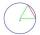Point on the circle is the end point of diameter and end point of chord length of radius. What angle between chord and diameter?
2. Arc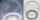The length of the circle is 41 amd arc length of the circle 9. What is the magnitude of the angle of this arc?
3. Disc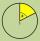Circumference of the disk is 78.5 cm. What is the circumference of the circular arc of 32° on the disc?
4. CancerOf the 80 people 50 people ill cancer. What percentage of people isn't ill?
5. Pipe cross sectionThe pipe has an outside diameter 1100 mm and the pipe wall is 100 mm thick. Calculate the cross section of this pipe.
6. FlowerbedIn the park there is a large circular flowerbed with a diameter of 12 m. Jakub circulated him ten times and the smaller Vojtoseven times. How many meters each went by and how many meters did Jakub run more than Vojta?
7. Wheels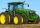Small tractor wheel with a diameter of 60 cm must be rotated 8 times to overcome some pathway. How many times must turn the big tractor wheel with a radius 60 cm to overcome the same distance?
8. Bridge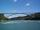The bridge arc has a span 235 m and height 3 m. Calculate the radius of the circle arc of this bridge.
9. Circle r,DCalculate the diameter and radius of the circle if it has length 52.45 cm.
10. Type of triangle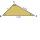How do I find the triangle type if the angle ratio is 2:3:7 ?
11. Obtuse angleWhich obtuse angle is creating clocks at 17:00?
12. SlopeFind the slope of the line: x=t and y=1+t.
13. EnrollmentThe enrollment at a local college increased 4% over last year's enrollment of 8548. Find the increase in enrollment (x1) and the current enrollment (x2).
14. DonutsFind how many donuts each student will receive if you share 126 donuts in a ratio of 1:5:8
15. ShotsDetermine the percentage rate of keeper interventions if from 32 shots doesn't caught four shots.
16. Arble bagA marble bag sold by Rachel's Marble Company contains 5 orange marbles for every 6 green marbles. If a bag has 35 orange marbles, how many green marbles does it contain?
17. 1.5 divided1.5 divided by 1 = w divided by 4